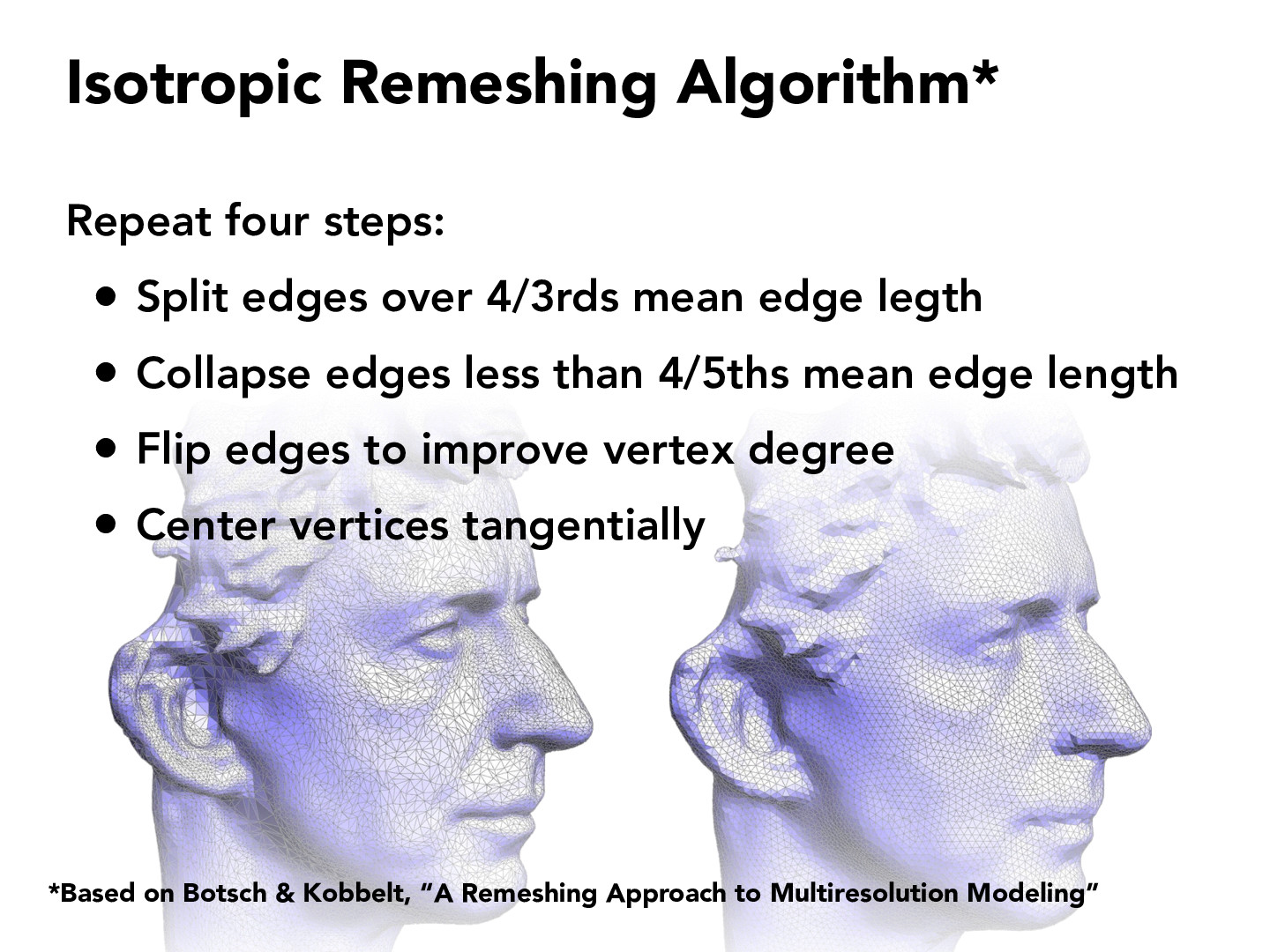Lecture 8: Meshes and Geometry Processing (78)ZiyeZhong

I just want to confirm that in the first two steps, the mean edge length means the average length of all edges in the geometry. By repeating these two steps, we are making the edge lengths more uniform. Is my understanding correct?hfan9

I believe you are correct. "Isotropy: having the same value when measured in different directions." The "isotropic" part of this algorithm's name suggests that the desired result is a mesh where the triangles are about the same size and shape. Since the three side lengths of a triangle determine a unique shape, forcing the edges to be roughly the same length should force all the triangles to be the same size and shape as well.

Something I found strange was this: suppose an edge is like 140% of the mean edge length. Since it's over 4/3 mean edge length, the first step would split it, and there'd be two 70% mean edge length edges (with a large number of edges, mean edge length. But then the second step would collapse both of these, since they're less than 4/5 (80%) mean edge length, so they'd be collapsed. In the end, the triangles of the original edge would both be collapse into a single edge. However, I think the misunderstanding came from the fact that all edges over 4/3 mean edge length are split. This would then change the mean edge length (making it smaller), changing the calculations for the second step.et-yao

Are 4/3 and 4/5 numbers that have been derived after some testing or something like that? Where'd they come from?

You must be enrolled in the course to comment